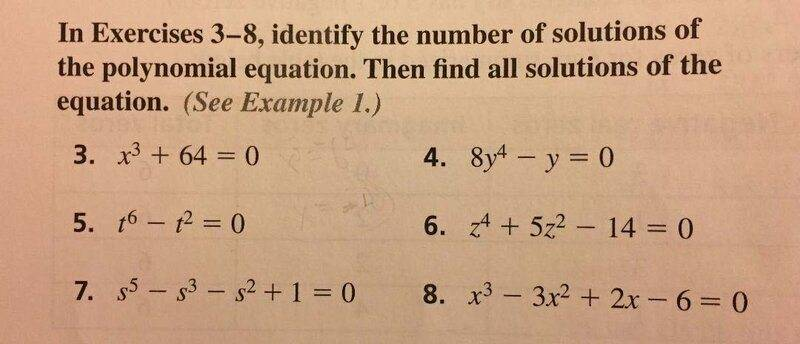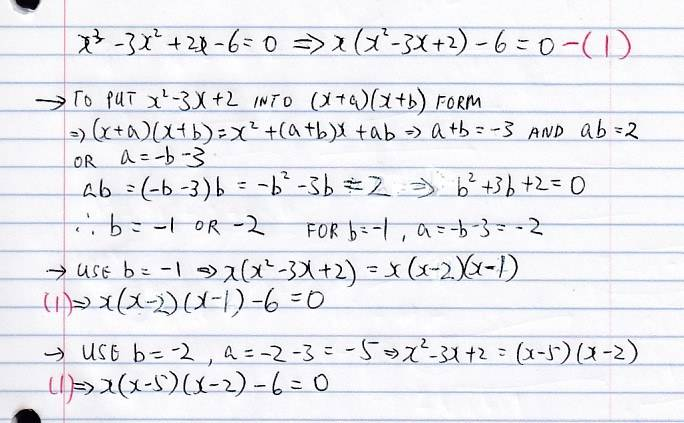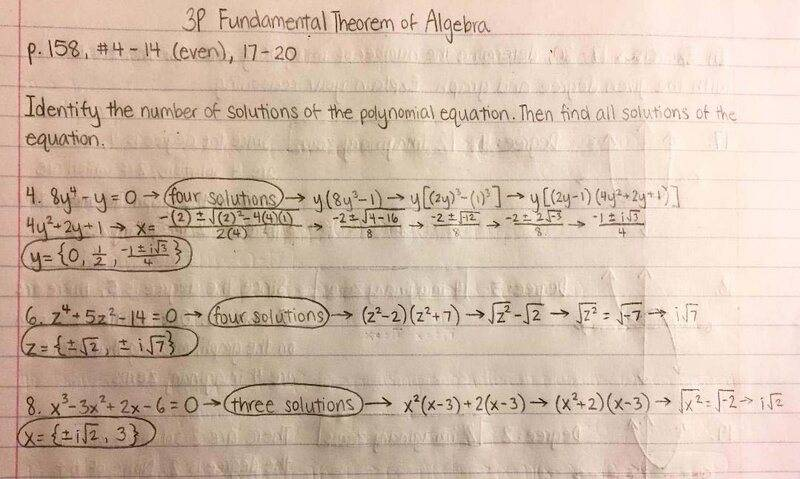Solving polynomial equations

Homework Statement:
Help with my grand daughter's homework
Old cat got his whiskers burned!!!
Relevant Equations:
don't know
I have a very urgent question, this is the problem. I have no idea how to solve this. I don't even know where to start. This is urgent, please at least tell me what is the name of this kind of problem so I can look it up.Specifically, # 4, 6 and 8.

I can guess #4 by dividing both sides by y to get 8y^3-1=0 or y^3 = 1/8 or y = 1/2.

I have no idea how to solve 6 and 8.

Thanks

Last edited:

PeroK
Homework Helper
Gold Member
2021 Award
As the problem says these questions involve "solving polynomial equations".

FactChecker
Gold Member
I can guess #4 by dividing both sides by y to get 8y^3-1=0 or y^3 = 1/8 or y = 1/2.
That's right. And that is not "guessing", that is how it should be done.
I have no idea how to solve 6 and 8.
For 6, set ##x=z^2## and factor ##x^2+5x-14##. That gives the values of ##x=z^2## and you can get the values of ##z## from it.
For 8, I don't know.

I looked online, for #6, I substitute u = z^2 to get u^2+5u-14=0 and solve u.

I still have no idea how to solve #8.

That's right. And that is not "guessing", that is how it should be done.

For 6, set ##x=z^2## and factor ##x^2+5x-14##. That gives the values of ##x=z^2## and you can get the values of ##z## from it.
For 8, I don't know.
I posted the same time as you. Yes, I have no idea on #8.

I am fighting Latex at the same time, how to you make equation in Latex form? I put ## x = z^2 ##, it doesn't come out.

PeroK
Homework Helper
Gold Member
2021 Award
For #8 just start guessing!

I posted the same time as you. Yes, I have no idea on #8.

I am fighting Latex at the same time, how to you make equation in Latex form? I put ## x = z^2 ##, it doesn't come out.
How come there is a delay reaction on Latex? When I first posted, it did not come out, then after a while, when I refresh, it turn to Latex form!!!

For #8, I simplified it, but still don't know how to solve it other than guessingAny suggestion?

thanks

PeroK
Homework Helper
Gold Member
2021 Award
For #8, I simplified it, but still don't know how to solve it other than guessing
View attachment 291277

Any suggestion?

thanks
Like I said, you should be able to guess one solution to get you started. There's nothing wrong with guessing.

I guess my grand daughter still the best. She figured out. This is her work she email me.She doesn't have to guess.

That's why she was the student of the year two years ago. I am so proud.

•oystein, hutchphd, OmCheeto and 1 other person
Office_Shredder
Staff Emeritus
Gold Member
2021 Award
For #8, the rational root test is a really good place to start. You just have to check if 1,2,3 and 6 are roots (also their negatives), find that 3 works, then you can factor (x-3) out and solve the remaining quadratic.

If there are no rational roots then this doesn't work, but if there are no rational roots of a cubic with integer coefficients you probably can't solve it anyway and it won't be on the homework :)

•mathwonk and PeroK
Mark44
Mentor
I can guess #4 by dividing both sides by y to get 8y^3-1=0 or y^3 = 1/8 or y = 1/2.
Dividing by the variable is not the right approach, because you will lose solutions. Since the original equation was of fourth degree, there are four solutions, one of which is y = 0. Besides this solution, and the solution you show, y = 1/2, there are two more solutions, both complex.
For #8 just start guessing!
That's one approach, but the approach used by the granddaughter is more clever. I first tried guessing, using synthetic division to test my guesses, but then noticed that factoring by grouping would have been much quicker.
##x^3 - 3x^2 + 2x - 6 = 0##
##\Rightarrow x^2(x - 3) + 2(x - 3) = 0##
##\Rightarrow (x^2 + 2)(x - 3) = 0##
From the last equation it can be seen that x = 3 is a solution, and there are two more (imaginary) solutions.

•sysprog, OmCheeto and Delta2
Mark44
Mentor
Maybe a bit over the top, but Cardano's formula can give you the roots for a 3rd degree polynomials:
Or overkill, in the context of the posted problem. I suspect that the textbook the granddaughter is using has some examples of "factoring by grouping," the technique I used in my earlier post.

•sysprog
Tom.G
And then there is:
https://math.vanderbilt.edu/schectex/courses/cubic/

But beware. It will keep you busy for a while with 64 operations.17 divides
20 square/cube
2 square root
2 cube root

epenguin
Homework Helper
Gold Member
For (8) spot that ##(x-3)## is an 'obvious' factor of the polynomial.

Which has been done.

•valenumr
osilmag
Gold Member
#3 is simple. ##-64^{1/3}=x##. That can be put into a calculator. It's obviously -4.

Last edited:
Mark44
Mentor
#3 is simple. ##-64^{1/3}=x##. That can be put into a calculator. It's obviously -4.

I posted the same time as you. Yes, I have no idea on #8.

I am fighting Latex at the same time, how to you make equation in Latex form? I put ## x = z^2 ##, it doesn't come out.
I guess my grand daughter still the best. She figured out. This is her work she email me.

View attachment 291279

She doesn't have to guess.

That's why she was the student of the year two years ago. I am so proud.
Yoir granddaughter's pemnmanship is extemely excellent ##-## up there with the finest of what we techies are apt to admiringly call 'girl-quality' ##-## I think that she could create excellent hand-written fonts all day long ##-## amazing ##-## beautiful . . .

mathwonk
Homework Helper
I believe Cardano's method used to be taught in high school, but before my birth. Consequently, the clearest explanation I have found is in the "elementary algebra" book by Euler, from which I adapted this presentation for bright young children, like your granddaughter, in a summer camp. All it requires is a good understanding of solving quadratics. This is from notes on my website, a little ways into them, below problem #13.
https://www.math.uga.edu/sites/default/files/inline-files/epsilon13.pdf

Next I want give Euler's explanation of how to solve cubic equations.
first he shows that any cubic equation can be transformed by a trick to change the cubic into one with no X^2 term. So it is only necessary to be able to solve cubics like this one:
X^3=pX+q. E.g.suppose that we have X^3=9X+28.

Then Euler explains that to solve this all we need to do is find two numbers u,v such that 3uv=9 and u^3+v^3=28. Then X=u+v will solve the cubic.
14. See if you can use Euler's method to solve X^3 = 9X + 28.

(Essentially this solution method was found apparently by Scipio del Ferro, and later Tartaglia, who explained it to Girolamo Cardano, who eventually published it. It is often called "Cardano's method".)

15. Try this one as well: X^3 = -18X + 19.

Solving cubics used to be taught in elementary algebra books, but when I went to high school, it was no longer done, and I think is not commonly done now. This is another reason to prefer the great old algebra books like those of Euler and LaGrange.

16. Show this method can be used on all cubics of form X^3 = pX + q.
I.e. i) show that one can always find numbers u,v such that u^3+v^3 = q, and 3uv = p.
(Hint: let A=u^3 and B=v^3. Then you know that A+B = q, and AB = p^3/27; why? Then show how to find A and B, and then tell how to find u and v.)

ii) Show that if X=u+v and if 3uv=p and u^3+v^3=q,thenX^3=pX +q.

The whole point of understanding quadratic equations is this. Write them like X^2-bX+c = 0, and then b is always the sum of the solutions and c is always their product.

Vice versa, whenever you are looking for two numbers and you already know their sum and their product, then you can always find the numbers as the solutions of a quadratic equation.

The whole point of solving cubics is this:
First we know from studying quadratics that we can always find two numbers whose sum and product are known.
Next Euler shows that to solve the cubic X^3 = pX + q, all you need is two numbers u,v such that u^3 +v^3 = q, and 3uv = p.
So we just need to find u and v. But it turns out we already know how to find their cubes, u^3 and v^3.
I.e. what do we know about the cubes of u,v? we know their sum u^3 + v^3 = q, and we know their product, since 3uv = p, so 27 u^3v^3 =p^3, hence u^3v^3 = p^3/27.

So since we know the sum and product of u^3 and v^3, we can find u^3 and v^3 by solving a quadratic! Then we can take cube roots to find u and v.

I.e. if r is a root of the quadratic t^2 - qt + (p^3/27), set u = cuberoot(r) and v = p/3u, and then u + v = X solves the cubic!

Unfortunately, it seems the given problem is not well suited to this method, but she might enjoy using it on #14 and #15 given here. Of course, the first step of Euler's method, substituting Y = X-1, or X = Y-1, and expanding yields the simpler equation Y^3 - Y -6 = 0, whose solutions are each one less than the solutions of the original equation, and 2 is an obvious solution here, but the other solutions are solutions of the quadratic Y^2 + 2Y + 3. A moral is perhaps that Cardano's formula is not always that useful, in comparison to the rational root theorem.

Last edited:
•jim mcnamara
For #8, the rational root test is a really good place to start. You just have to check if 1,2,3 and 6 are roots (also their negatives), find that 3 works, then you can factor (x-3) out and solve the remaining quadratic.

If there are no rational roots then this doesn't work, but if there are no rational roots of a cubic with integer coefficients you probably can't solve it anyway and it won't be on the homework :)
Integer fractions are rational... But for me the x = 3 root just sort of popped out.

Integer fractions are rational... But for me the x = 3 root just sort of popped out.
Oh geez, I feel silly, the first coefficient is 1DaveE
•Wow, that's good, @DaveE ##-## I especially liked "• Exact general solution is hopeless" ##-## as something weakly indicative of the insolubility of the quintic.